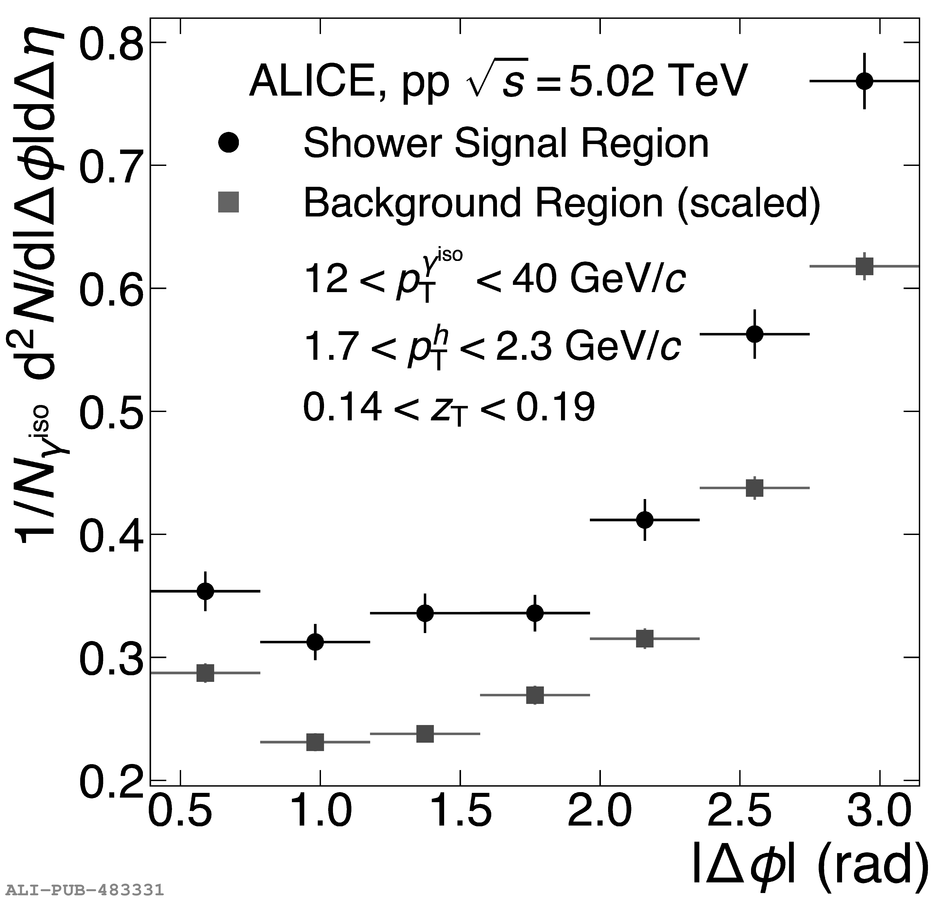# Figure 3

 $\gammaiso$--hadron signal region (black circles) and background region (grey squares) correlations in pp collisions at \sqrts~= 5.02 TeV as measured by the ALICE detector. The shower signal region photons correspond to isolated clusters with \lambdasquare $0.4$. The vertical bars represent statistical uncertainty only. The horizontal bars represent the bin width in $|\Delta\varphi|$. The background correlation is subtracted from the signal correlation according to the numerator in Eq. \ref{Corr_Subtraction}.